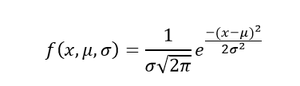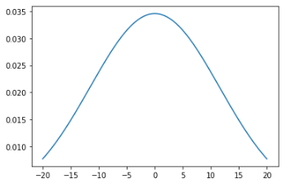Related Articles

# How to plot a normal distribution with Matplotlib in Python ?

• Last Updated : 03 Jan, 2021

Prerequisites:

Normal Distribution is a probability function used in statistics that tells about how the data values are distributed. It is the most important probability distribution function used in statistics because of its advantages in real case scenarios. For example, the height of the population, shoe size, IQ level, rolling a die, and many more.

The probability density function of normal or Gaussian distribution is given by:Probability Density Function

Where, x is the variable, mu is the mean, and sigma standard deviation

### Modules Needed

• Matplotlib is python’s data visualization library which is widely used for the purpose of data visualization.
• Numpy is a general-purpose array-processing package. It provides a high-performance multidimensional array object, and tools for working with these arrays. It is the fundamental package for scientific computing with Python.
• Scipy is a python library that is useful in solving many mathematical equations and algorithms.
• Statistics module provides functions for calculating mathematical statistics of numeric data.

### Functions used

• To calculate mean of the data

Syntax:

`mean(data)`
• To calculate standard deviation of the data

Syntax:

`stdev(data)`
• To calculate normal probability density of the data norm.pdf is used, it refers to the normal probability density function which is a module in scipy library that uses the above probability density function to calculate the value.

Syntax:

norm.pdf(Data, loc, scale)

Here, loc parameter is also known as the mean and the scale parameter is also known as standard deviation.

### Approach

• Import module
• Create data
• Calculate mean and deviation
• Calculate normal probability density
• Plot using above calculated values
• Display plot

Below is the implementation.

## Python3

 `import` `numpy as np``import` `matplotlib.pyplot as plt``from` `scipy.stats ``import` `norm``import` `statistics`` ` `# Plot between -10 and 10 with .001 steps.``x_axis ``=` `np.arange(``-``20``, ``20``, ``0.01``)`` ` `# Calculating mean and standard deviation``mean ``=` `statistics.mean(x_axis)``sd ``=` `statistics.stdev(x_axis)`` ` `plt.plot(x_axis, norm.pdf(x_axis, mean, sd))``plt.show()`

Output:The output of above code

Attention geek! Strengthen your foundations with the Python Programming Foundation Course and learn the basics.

To begin with, your interview preparations Enhance your Data Structures concepts with the Python DS Course. And to begin with your Machine Learning Journey, join the Machine Learning – Basic Level Course

My Personal Notes arrow_drop_up